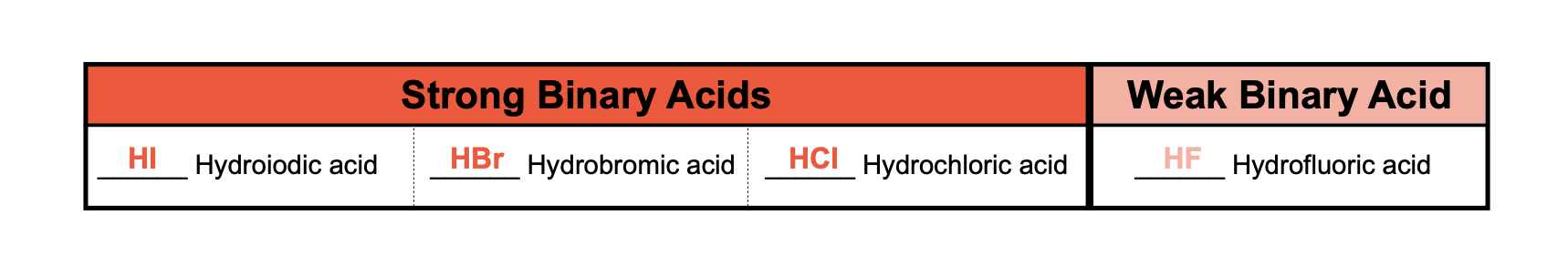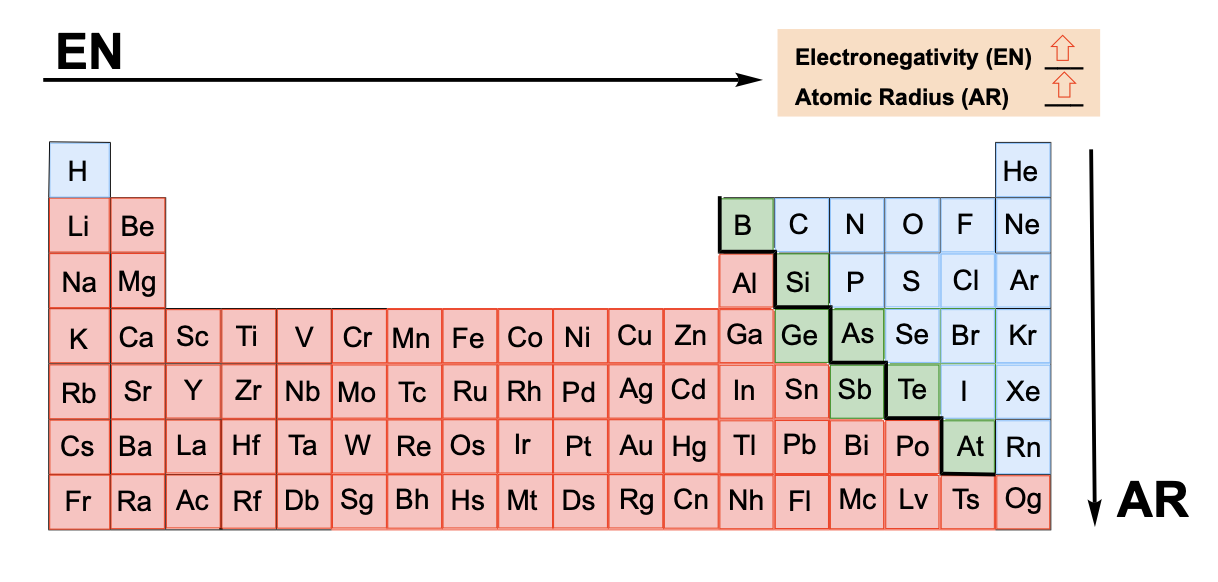Clutch Prep is now a part of Pearson
Ch.15 - Acid and Base EquilibriumWorksheetSee all chapters

# Binary Acids

See all sections
Sections
Acids Introduction
Bases Introduction
Binary Acids
Oxyacids
Bases
Amphoteric Species
Arrhenius Acids and Bases
Bronsted-Lowry Acids and Bases
Lewis Acids and Bases
The pH Scale
Auto-Ionization
Ka and Kb
pH of Strong Acids and Bases
Ionic Salts
pH of Weak Acids
pH of Weak Bases
Diprotic Acids and Bases
Diprotic Acids and Bases Calculations
Triprotic Acids and Bases
Triprotic Acids and Bases Calculations
Conjugate Acids and Bases

Binary Acids are the first class of covalent acids

###### Binary Acids

Concept #1: Binary Acids represent covalent compounds containing a hydrogen ion and a non-oxygen anion.

Example #1: Which of the following represents the possible structure of a binary acid?

a) HCNO                                  b) BaCl­2                                    c) HF                                        d) LiH

Concept #2: A majority of binary acids are weak with exception to those containing some of the halogens.Example #2: The following represent aqueous acid solutions. Identify the strong acid, weak acid and weakest acid.

Concept #3: The strength of binary acids is based on differences in electronegativity and atomic radius.

Recall, as you move to the top right of the periodic table the electronegativity increases and the atomic radius decreases.Example #3: Which is the weakest acid from the following?

a) HF                            b) HCl                           c) HI                             e) HBr                           d) All are equal.

Example #4: Which is the weakest acid from the following?

a) H2Se                         b) HF                            c) H2Te                         e) H2S                          d) All are equal

Practice: Which of the following acids would be classified as the strongest?

a) CH4                          b) H2Te                         c) H2S                           d) PH3                          e) BH3